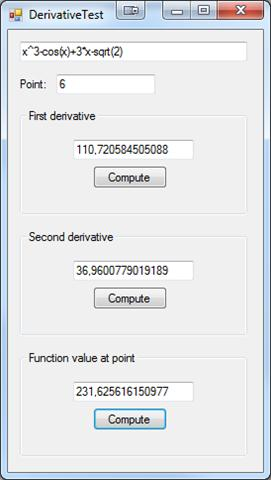### Features And Algorithms

This library uses numerical calculator library to compute derivatives so can handle same functions.

Mathematical formulas used to compute derivatives are transformations of Taylor's theorem. Both formulas are accurate to O(h^4) where h is the step used in computation (default 0.001).

### Usage

`string formula = "x^3-cos(x)+3*x-sqrt(2)";double point = 2.2;Derivative derivative = new Derivative(formula);double resultFirstDerivative = derivative.ComputeDerivative(point);double resultSecondDerivative = derivative.ComputeDerivativeBis(point);double resultFunctionValue = derivative.ComputeFunctionAtPoint(point);`

### Example application